# Convective Heat Transfer in an Annulus of Concentric and Eccentric Cylinders with an Inner Rotating Cylinder

Convective Heat Transfer in an Annulus of Concentric and Eccentric Cylinders with an Inner Rotating Cylinder
Mechanical Engineering Department, University of Anbar, Ramadi 31001, Iraq

The Renewable Energy Research Centre, University of Anbar, Ramadi 31001, Iraq

Corresponding Author Email:
eng.ajsh2006@uoanbar.edu.iq
Page:
61-72
|
DOI:
https://doi.org/10.18280/ijht.390107
13 October 2020
|
Accepted:
20 January 2021
|
Published:
28 February 2021
| Citation

OPEN ACCESS

Abstract:

This numerical study details the engine oil flow behaviour of convective heat transfer through a concentric and an eccentric annulus formed between a heated inner rotating cylinder and a cooled outer stationary cylinder. The three-dimensional governing equations for continuity, momentum, and energy have been solved using a finite volume method (FVM). The computational simulations are developed using well-known commercial software ANSYS-FLUENT. Besides this, a sensitivity analysis is executed via response surface methodology (RSM) using the statistical software of Design-Expert 12 to predict the optimum design that improves the hydrothermal performance in an eccentric annular cylinder. The findings are reported for numerical calculations of the moment coefficient (Cmc), surface temperature (Ts) of the inner rotating cylinder and Nusselt number (Nu) for a range of values of (0.25 - 0.75) aspect ratio (R*), (0.5 - 2.5 cm) eccentricity (E), (0° – 180°) inclination angle of the inner rotating cylinder (θ) and a wide range of Taylor number (Ta) of (2 – 4.5×104). In the case of a concentric annular cylinder, the averaged Nusselt number increases by increasing the Taylor number and decreasing the aspect ratio. Also, increasing the Taylor number and decreasing the aspect ratio causes a gradual decrease in the moment coefficient and surface temperature of the inner rotating cylinder. Whereas in the case of the eccentric annular cylinder, the maximum averaged Nusselt number and minimum surface temperature with the moderate value of moment coefficient are obtained at the optimum case of 2.5 cm eccentricity and 180° inclination angle, which are considered the optimum-estimated parameters of the eccentric annular gap. The influences of these physical parameters are graphically depicted in terms of velocity contours and temperature fields. Therefore, the rotation of the inner cylinder is shown to have a significant impact on the thermal-flow field of confined fluid.

Keywords:

concentric and eccentric annular cylinder, rotating internal cylinder, rotational moment coefficient, Taylor-Couette flow, response surface methodology

1. Introduction

The patterns of fluid flow and characteristics of convective heat transfer in an annular gap between concentric and eccentric annular cylinders with the absence of streamwise flow have increasingly received remarkable attention. There are many practical applications of annular pipe flows, such as rotational electrical machines, in oil and gas production, fluid viscometers, electrochemical devices, tribology, hydraulic equipment and chemical reactors . Therefore, a deep understanding of the flow behaviour in an annular gap with a stationary outer wall and a rotating inner wall should be given within the wide scope of fluid flows through annular regions.

In an accomplished contribution to the literature of fluid flow behaviour between coaxial smooth cylinders, the first experimental measurements with two concentric cylinders by Couette [2, 3] and also by Mallock [4, 5] pointed out that the critical speed of cylinders was much lower in the case of the internal rotating cylinder and the outer stationary cylinder than vice versa. Later, the theoretical and experimental investigations by Taylor  has pioneered an understanding of the stability criterion of azimuthal Couette flow with viscous force influence that acting on the fluid flow. Taylor was one of the pioneers in employing the theory of linear stability to solve this issue. He was capable of obtaining a brilliant agreement of theory with experiments for the flow instability between two coaxial rotating cylinders [7, 8]. He outlined the presence of a critical rotation speed when the flow under that critical speed is steady-state and laminar (known as Couette flow). The fluid streamlines, in this case, are described as annular and centred on the rotational speed axis. Taylor discovered the appearance of secondary flow in a toric form around the inner cylinder, which arises beyond the critical rotation speed, i.e., after the first onset of instability. These vortices are counter-rotative and connected by pairs [9, 10]. Taylor was able to determine the critical value of rotational speed (Tac = 1708) according to his experimental and theoretical results. In addition, from both the experimental and the theoretical perspective, a large group of researchers agreed with this critical value of Tyler number, for example, Bjorklund and Kays Tac = 1770-1994 , Tachibana et al. Tac = 1730-3000 , Becker and Kaye Tac = 1994 . In the physical and engineering communities, it is well-known that fluid flow through two independently concentric rotating cylinders is named in the term Taylor-Couette flow after British physicist Taylor  and French physicist Mallock [4, 5]. As a consequence of Taylor's pioneering study, these vortices are usually called "Taylor's vortices".

When the streams of forced circulation flow are low somewhat, free convective heat transfer inherently arises in the annular gap due to the dominant buoyancy force. Guo and Zhang  recognized that for a Rayleigh number less than 104, the impact of free convection is negligible. Whilst with increasing Rayleigh number beyond 104, in this case, fluid flow within annulus depends on the parameter Gr/Re2, which represents the significance of free convection relative to forced convection. The survey of previous publications highlighted that many studies addressed the free convection heat transfer in the concentric annulus see for example [15-19]. The influence of Taylor-Couette flow structures on heat transfer was experimentally and numerically studied. These efforts were surveyed by Maron and Cohen  and more recently by Fénot et al. .

From the early studies in this field, Luke  and Hoseasou  individually investigated convection heat transfer through the rotating surface of the inner cylinder to the stationary surface of the outer cylinder (rotor and stator surfaces of the electrical motor) with approximately zero axial flow. Their experimental studies on the cooling of the electrical machine had no obvious results and conclusions due to the issues with dimensions and set-up of apparatus used. In the period between the 1950s and 1960s, Gazley Jr [24, 25], Bjorklund and Kays , Tachibana et al. [12, 26], and Aoki et al.  reported experimental studies, which addressed convective heat transfer in the annulus with rotating inner cylinder. Gazley Jr [24, 25] conducted a series of experiments on the electric motors with an inner rotating cylinder, which was heated by means of a resistance-heating coil, and a stationary outer cylinder was cooled by a water jacket to quantify the rate of heat transfer. He also examined the impact of different combinations of longitudinal flow and speeds of rotation on the heat transfer rate. Whereas, Bjorklund and Kays  tested four different annular gaps with six different ratios of outer cylinder speed to inner cylinder speed and zero axial fluid flow in these annular gaps. They correlated their experimental data in terms of heat transfer rate (Nusselt number) for these annular gaps and the speed ratio over a wide range of Taylor number. Becker and Kaye [13, 28] presented a systematically experimental investigation to study convective heat transfer in an annular cylinder involving an internal rotating heated cylinder and an external stationary cooled cylinder to mimic the air gap of a rotating electrical machine. They also concerned the effect of presenting temperature gradient and heat transfer on annular flow structure at a wide range of longitudinal flow rates and rotation speeds. They were able to correlate their heat transfer findings with those of past publications in terms of Taylor number and Reynolds number in fairly simple formulas. In the same year, Becker and Kaye  analytically revealed that heating of the inner rotating cylinder stabilizes the annular flow, whilst heating of the outer stationary cylinder destabilizes the annular flow. Ball and co-authors published numerical  and experimental  studies to evaluate the characteristics of heat transfer and flow patterns, which develop around an internal rotating cylinder of two concentric cylinders. The inner rotating cylinder was heated whereas the outer stationary cylinder was cooled. Their results illustrated that there is a strong interdependence between the mechanism of heat transfer and the structure of the secondary flows that arise in the annulus. Also, qualitative observation of the secondary flow characteristics of the rotating system was shown using smoke visualization techniques to provide a qualitative overview of the transition from a buoyancy-dominated flow regime to that dominated by rotation flow.

Chart and Hsu  have simulated turbulent mixed convection of air in a concentric annular cylinder with a cooled external cylinder and a heated rotating internal cylinder. For the centrifugal configurations, the results illustrated that the averaged Nusselt number increases with an increase in the Rayleigh number, however, it decreases with an increase in the Reynolds number or radius ratio. In their experimental research, Lee and Minkowycz  demonstrated that the increased axial Reynolds number has a negligible impact on flow and heat transfer, provided the respective values of rotation velocity and axial flow (103 < Ta < 2×107 and 52 < Re < 1000). A 3D numerical investigation was executed to study the impact of the inner pipe rotation speed (100, 200, 300, 400 and 500 rpm) and eccentricity ratio (changing the centre of internal pipe from 0 to 40 mm from the centre of the external pipe) on the hydrothermal performance of double-pipe heat exchanger by Ali et al. . The numerical results elucidated that there was a significant enhancement in heat transfer by 223% when changing the eccentricity up to 40 mm with the inner pipe rotation of 500 rpm, meanwhile, the pressure-drop penalty through the heat exchanger increased by 53%. Abou-Ziyan et al.  and Yassin et al.  executed an experimental study to enhance forced convection heat transfer in a concentric annular pipe with an internal finned pipe under rotating conditions. The combined effects of using a passive technique of heat transfer enhancement (interrupted helical fins) and active technique (rotating internal pipe) on the heat transfer and pressure drop characteristics in annular passage augment Nusselt number more than seven times compared with the Nusselt number of smooth stationary annular pipe.

This article spotlights the engine oil flow behaviour and convective heat transfer in the annular gap of the concentric and eccentric cylinder with an inner rotating cylinder. Different parameters such as aspect ratio (R*) and the rotation speed of the internal cylinder (Ω), are numerically addressed. The innovation of this investigation is a sensitivity analysis is executed via response surface methodology (RSM) using the statistical software of Design-Expert along with ANSYS-FLUENT to predict the optimum design that improves the hydrothermal behaviour and performance in an eccentric annular cylinder. Thus, the changing the eccentricity of the inner rotating cylinder (E), i.e. the r-axis locations of the inner cylinder, with different rotation angles (θ) is considered by considering two input independent factors, 21 experimental runs and five levels. These experimental runs are then carried out as a function of these input variables for gaining the set of designing conditions that optimize the hydrothermal characteristics of the eccentric annular cylinder. Results are arranged in three stages of this numerical study. In the first stage, the validation of the numerical model is achieved via studying free convection heat transfer as a standard case with altering aspect ratio between these coaxial cylinders and then compared results with previously published data. In the second stage, temperature distributions, oil flow patterns, average Nusselt number and moment coefficient are presented with a wide range of rotation speeds of the concentric internal cylinder. A third stage is concentrated on the optimum analyzation influence of annulus eccentricity with several inclination angles to obtain the desired system performance.

2. Computational Approaches

2.1 Problem description

In the present study, the numerical model involves concentric and eccentric cylinders in a z-horizontal position with an inner rotating cylinder. The rotating inner cylinder of the radius (Rin) is heated using a constant heat flux whereas the outer stationary cylinder of the radius (Rout) is kept at room temperature (Tc = 25°C) for both concentric and eccentric cases. This annulus is covered by fixed and adiabatic end-plates. Different parameters such as aspect ratio (R* = Rin/Rout) and the speed of the internal cylinder are considered. The angular velocity of the rotating inner cylinder ranges from 0 rpm to 3000 rpm in 7 increments with 500 rpm for each increment (Ta = 2 – 4.5×104). Three aspect ratios of R* = 0.25, 0.5 and 0.75 are considered, as shown in Figure 1. Engine oil is employed as a working fluid that fills the annular gap between the outer and inner cylinder to investigate the behaviour and the thermal-hydraulic performance of this kind of fluid. Furthermore, the effect of eccentricity, E, of the rotating inner cylinder is optimized using values of (E = 0.5, 1, 1.5, 2 and 2.5 cm) with different rotational angles (θ = 0°, 45°, 90°, 135° and 180°), as shown in Figure 2.

## 1.pngFigure 1. Different configurations of concentric annular cylinder with different aspect ratios (R*)

## 2.pngFigure 2. Eccentric annular cylinder with different values of E and θ at R* = 0.25

2.2 Governing equations and assumptions

The thermal-hydraulic characteristics of both concentric and eccentric horizontal annular cylinders are evaluated using ANSYS FLUENT-CFD for different aspect ratios with the rotating inner cylinder. The main assumptions of this study are three-dimensional, steady-state, viscous engine oil, single-phase, incompressible and laminar fluid flow. The rotational speed of the internal cylinder is uniform that often applied in practical and industrial applications and assumed in many published papers [27-38]. Constant thermophysical properties of engine oil is presumed.

Continuity equation:

Generally, the differential equation of mass conservation for steady-state incompressible fluid flow of the cylindrical polar coordinate system is defined as [36, 37],

$\frac{1}{r} \frac{\partial}{\partial r}\left(r u_{r}\right)+\frac{1}{r} \frac{\partial}{\partial \theta}\left(u_{\theta}\right)+\frac{\partial}{\partial z}\left(u_{z}\right)=0$     (1)

An axial velocity uθ, a radial velocity uθ, and a circumferential velocity uθ, which is positive counterclockwise, i.e. in the direction of increasing θ, are the three independent velocity components. Accordingly, in this case, there is no axial and radial flow (uz = 0 and ur = 0), the continuity equation is reduced to Eq. (2).

$\frac{\partial u_{\theta}}{\partial \theta}=0$     (2)

Momentum equations:

Similarly, the radial and tangential and components of the Navier-Stokes equations are reduced to Eqns. (3), (4) and (5) in the cylindrical (r, θ and z) coordinates [36, 37]:

$r$ -component: $-\frac{\rho u_{\theta}^{2}}{r}=-\frac{d p}{d r}$     (3)

$\theta$ -component: $\mu\left[\frac{d}{d r}\left(\frac{1}{r} \frac{d}{d r}\left(r u_{\theta}\right)\right)\right]=0$     (4)

$z$ -component: $-\frac{d p}{d z}-\rho g=0$     (5)

If end effects are neglected, then uθ(r, z) does not depend on z. Thus, uθ = uθ(r). Furthermore, due to centrifugal forces, the r-component provides the radial pressure distribution and the z-component gives the axial pressure distribution due to gravitational forces .

Energy equation:

$\left(\frac{u_{\theta}}{r} \frac{\partial T}{\partial \theta}\right)=\alpha\left[\left(\frac{1}{r} \frac{\partial}{\partial r}\left(r \frac{\partial T}{\partial r}\right)\right)+\frac{1}{r^{2}}\left(\frac{\partial^{2} T}{\partial \theta^{2}}\right)+\frac{\partial^{2} T}{\partial z^{2}}\right]$     (6)

The hydraulic diameter (Dh) for the annular cylinder is defined as ,

$D_{h}=\frac{4 A_{c}}{P_{m}}=\frac{4 \times \pi\left(R_{\text {out }}^{2}-R_{\text {in }}^{2}\right)}{2 \pi\left(R_{\text {out }}+R_{\text {in }}\right)}=2\left(R_{\text {out }}-R_{\text {in }}\right)$     (7)

whereas the annular gap width (e) is expressed as ,

$e=\left(R_{\text {out }}-R_{\text {in }}\right)$     (8)

Thus, the definition of hydraulic diameter (Dh) can be rearranged as,

$D_{h}=2 \times e=2\left(R_{\text {out }}-R_{\text {in }}\right)$     (9)

As mentioned before, the aspect ratio (R*) of the annular cylinder is expressed as,

$R^{*}=\frac{R_{\text {in }}}{R_{\text {out }}}$     (10)

For the rotational annular cylinder, the Taylor number (Ta) is usually employed instead of the Reynolds number. Taylor number may be defined as the ratio between centrifugal and viscous forces ,

$T a=\frac{\Omega^{2} R_{i n}\left(R_{\text {out }}-R_{\text {in }}\right)^{3}}{v^{2}}=\frac{\Omega^{2} R_{\text {in }}\left(\frac{D_{h}}{2}\right)^{3}}{v^{2}}$$=\frac{\Omega^{2} R_{i n}(e)^{3}}{v^{2}}$     (11)

where, Ω is the angular speed of rotation of the inner cylinder (rad/s) and ν is the kinematic viscosity (m2/s).

Typically, with using high viscous fluid like engine oil, viscous forces are much higher than inertia forces; thus, the need to calculate the power required to overcome a rotating cylinder's frictional drag is practically essential . This includes flow in an annulus in cylindrical coordinates, as shown in Figure 3, and the Navier-Stokes equations can be solved precisely by analytical techniques, subject to several important assumptions with appropriate boundary conditions (at $r=R_{i n}, u_{(\theta)}=\Omega \times R_{i n},$ and at $r=R_{\text {outs }} u_{(\theta)}=0$) to provide the velocity distribution as a function of radius and the torque on the inner and outer cylinders [36, 39, 40]:

$u_{(\theta)}=\frac{-\Omega R_{i n}^{2}}{R_{o u t}^{2}-R_{i n}^{2}} r+\frac{\Omega R_{i n}^{2} R_{o u t}^{2}}{R_{o u t}^{2}-R_{i n}^{2}} \frac{1}{r}$     (12)

The viscous shear stress at r = Rin is given by

$\left.\tau\right|_{r=R_{\text {in }}}=\mu \frac{r \partial\left(u_{\theta} / r\right)}{\partial r}=-2 \mu \frac{\Omega R_{\text {out }}^{2}}{R_{\text {out }}^{2}-R_{\text {in }}^{2}}$     (13)

For laminar flow, the torque coefficient can be deduced from the linear theory as a function of angular velocity. The torque (Tr) per unit annular length L, which is the torque transmitted to an outer cylinder as a function of the angular velocity of the inner cylinder, is equal to (τAsRin /L) for the circumferential flow within the concentric annular cylinder. The cylinder surface area is (2πRinL), therefore the torque per unit length is [36, 39, 40],

$T_{r}=\frac{\tau\left(2 \pi R_{\text {in }} L\right) R_{\text {in }}}{L}=-4 \pi \mu \frac{\Omega R_{\text {out }}^{2} R_{\text {in }}^{2}}{R_{\text {out }}^{2}-R_{\text {in }}^{2}}$      (14)

Now, it is possible to express the moment coefficient, Cmc, for a rotating cylinder by [39, 40]:

$C_{m c}=\frac{T_{r}}{\frac{1}{2} \pi \rho \Omega^{2} R_{i n}^{4} L}=\frac{8 R_{o u t}^{2}}{R_{i n}\left(R_{\text {out }}+R_{\text {in }}\right)} R e_{\theta}^{-1}$      (15)

where, Reθ is the rotational Reynolds number, also known as a Couette Reynolds number, which usually introduces in issues of a rotating viscous fluid and represents the ratio between inertial forces and viscous forces, based on the width of the annular gap defined as ,

$R e_{\theta}=\frac{\Omega R_{i n}\left(R_{o u t}-R_{i n}\right)}{v}$      (16)

The average Nusselt number $(\overline{N u})$ is considered based on the total surface area of the hot internal cylinder (Ain) :

$\overline{N u}=\frac{\bar{h} D_{h}}{k_{f}}$      (17)

where, kf is the fluid thermal conductivity and $\bar{h}$ is the average heat transfer coefficient for the rotating inner cylinder and determined by the following :

$\bar{h}=\frac{q^{\prime \prime}}{A_{i n}\left(T_{S}-T_{m}\right)}$      (18)

where, Ts and Tm are the surface temperature and the mean fluid temperature, respectively, and q” is the subjected heat flux on the inner cylinder.

2.3 Boundary conditions

The main boundary condition is performed for concentric and eccentric annular cylinders, as shown in Figure 3, for simplifying the computational solution. The stationary external cylinder is at constant temperature (Tc = 25°C) with zero angular velocity (no‐slip condition, Ωout = 0). While the uniform constant heat flux (q” = 5000 W/m2) is applied along the internal cylinder with/without angular velocity (Ωin = 0, 500, 1000, 1500, 2000, 2500 and 3000 rpm). The end-plates of the annulus are assumed to be isolated ($\frac{\partial T}{\partial n}=0$) and a no‐slip condition (Ω = 0). Engine oil is filled the annular gap between the internal and external cylinder (see  Table 1 for thermophysical properties of unused engine oil ).

Table 1. Thermophysical properties of unused engine oil at 25°C 

 Property 25°C  Density, ρ (kg/m³) 885.075 Specific heat, Cp (J/kg.K) 1901.75 Thermal conductivity, k (W/m.K) 0.14485 Dynamic viscosity, μ (Ns/m²) 0.68248 Thermal expansion coefficient, β (1/K) 0.00070 Prandtl number, Pr (-) 8960.28

## 3.pngFigure 3. Boundary conditions for both concentric and eccentric annular cylinder

2.4 Computational implementation procedure

The geometry of concentric and eccentric annular cylinder is modelled. Then, the physical domain and grid generation of concentric and eccentric annular horizontal cylinders are conducted by ANSYS FLUENT-CFD , as shown in Figure 4. The quadrilateral face shape and hexahedral grid are applied for both concentric and eccentric cylinder. An intensive grid structure is carried out at the internal and external surfaces of the cylinders leading to a better match of the isothermal and engine oil velocity gradients inside the annular gap compared with a coarse grid structure. The assumptions, boundary conditions and governing equations are employed to solve the problem of the studied computational domain. This software is adopted for solving the laminar convection heat transfer of oil flow with/without rotating inner cylinder using the finite volume method (FVM) . For the stationary case (no rotation of inner cylinder), the Second-Order upwind with SIMPLE (Semi Implicit Method for Pressure Linked Equations) algorithm scheme is chosen for the pressure-velocity coupling, the momentum and energy equations. However, the Coupled Scheme is applied for inner rotating cylinder convergence. The pressure equation is discretized utilizing the PRESTO! Scheme. The computational solution is convergent as the residual sum for the momentum and continuity and energy equations less than 10-6.

## 4.png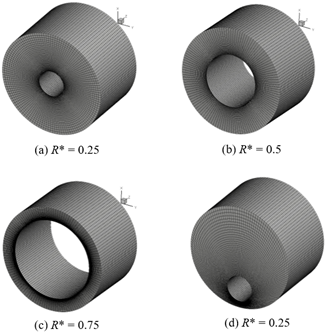Figure 4. The simulated average Nusselt number ($\overline{N u}$) and the surface temperature (Ts) of rotating inner cylinder using different numbers of cells

3. Results and Discussion

3.1 Grid independent test and validation

The grid Independent Test (GIT) is firstly executed to obtain an appropriate mesh density that provides sufficiently accurate numerical results. Numerical simulations were performed using engine oil flowing through an annular gap. Six different mesh resolutions were examined at the angular rotation speed of the concentric inner cylinder of 0 rpm and 3000 rpm with the stationary outer cylinder at 0.5 aspect ratio. The numbers of cells that utilized in the present computational calculations are GIT 1= 27×103, GIT 2= 59×103, GIT 3= 108×103, GIT 4= 138×103, GIT 5= 180×103 and GIT 6= 250×103 cells as illustrated in Figure 5. The numerical error is proportional to the square of the mesh size as applied to the exact solution. To boost the precision of the numerical data collected, the mesh sizes should be decreased. In modern CFD setup, mesh generation and gridding have been very refined. High-density gridding wraps around the circular edges, catching significant near-wall data. The main sources and causes of the numerical error results illustrating in Figure 5 are the results of coarse mesh resolutions, thus the numerical errors can be reduced via the utilization of fine mesh. It can be noticed from Figure 5, the average Nusselt number $(\overline{N u})$ and the surface temperature (Ts) of the rotating inner cylinder for different grid resolutions are all in very good agreement, in spite of utilizing different mesh resolutions. Also, the resulted numerical data are listed in Table 2. As depicted in this table, computational simulation of GIT 4= 138×103 cells yields $\overline{N u}$= 4.39 and 31.75 and Ts = 106.1°C and 36.2°C at Ω = 0 and 3000 rpm, respectively. In comparison with that of GIT 5= 180×103 cells, it only yields a slight deviation of less than 0.8% and 0.6% for $\overline{N u}$ and Ts, respectively, as calculated. Thus, the numerical results of GIT 4 with approximately 140,000 cells are considered adequate to achieve accurate results for this investigation and thus used hereafter.

Table 2. Convergence study for the average Nusselt number ($\overline{N u}$) and the surface temperature (Ts) of rotating an inner cylinder with 0.5 aspect ratio

 Mesh Symbol Number of Cells×103 $\overline{N u}$ Ts $\overline{N u}$ Ts 0 rpm 0 rpm 3000 rpm 3000 rpm GIT 1 27 4.55 103.28 20.58 41.99 GIT 2 59 4.47 104.65 27.77 37.71 GIT 3 108 4.42 105.60 31.28 36.40 GIT 4 138 4.39 106.10 31.75 36.19 GIT 5 180 4.36 106.65 32.00 36.08 GIT 6 250 4.33 107.00 32.25 36.00

## 5.pngFigure 5. The simulated average Nusselt number ($\overline{N u}$) and the surface temperature (Ts) of rotating inner cylinder using different numbers of cells

In order to validate the adopted numerical procedure, verifications of the predicted computational data for free convection heat transfer in a concentric annulus are performed. Figure 6 exhibits the heat flux from the inner and outer cylinder surfaces for one of the natural convection simulations in a concentric annulus at Rayleigh number = 4.95×104, Prandtl number = 0.707 and aspect ratio = 0.5. The current numerical results shown in Figure 6 are in good agreement with Kuehn and Goldstein's experimental findings .

Another comparison was made under the same experimental conditions of Kuehn and Goldstein  for both concentric and eccentric annular cylinder. Figure 7 (a) presents the numerical temperature distribution of concentric annular cylinder whilst Figure 7 (b) - (d) displays the current numerical temperature distribution of eccentric annular cylinder with that of experimental temperature distribution at three different positions. Generally, features of the temperature distribution (isothermal contours) for both concentric and eccentric annular cylinder resulted from the numerical analysis reasonably resemble with these gained from experiments with a slight deviation in the temperature distribution between them. This deviation in the numerical result may be traced back to the constant thermophysical properties of the working fluid that have been assumed or because of the finite number of cells.

## 6.png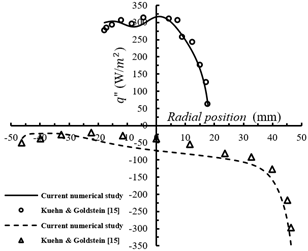Figure 6. The heat flux from the inner and outer cylinder surfaces for the current numerical data and previous experimental results for Kuehn & Goldstein 

The behaviour of fluid movement due to the free convection circulation in the concentric annular gap is numerically studied using three-dimensional CFD simulations. Figure 8 depicts contours of temperature, velocity magnitude and relevant vectors across a cross-sectional plane corresponding to the aspect ratios (R* = 0.75, 0.5 and 0.25). In this section, the value of the Prandtl number is fixed at Pr = 8960.28 to observe the impact of the aspect ratio on the flow behaviour and the heat transfer characteristics within the annular cylinder.

Figure 8 displays the plots of temperature distribution fields (right) and velocity contours with vectors (left) for three various values of aspect ratio. The results of numerical simulations at all values of aspect ratio illustrate that the buoyant plume above the heated inner cylinder impinges upon the outer cylinder at the top owing to the buoyancy force effect. Thus, this indicates that natural convection is the dominant fashion of heat transfer mechanism under this condition. Due to the natural convection circulation, the isotherms of temperature distribution move upward and large plumes go out around the top of the outer cylinder mentioning the existent of the strong thermal gradient at this zone. Besides, the plots of velocity vectors show the main flow is generated at the upper half of the concentric annular cylinder where the centre of the main formed vortex is located at this zone. As can be noticed from velocity contours, with increasing the aspect ratio from 0.25 to 0.75, the size of the formed vortex also rises. The temperature contours are significantly distorted because of the strong intensity of the convection effect, leading to stable stratification of the isotherms. The vortex slightly moves upward due to the high rotational flow resulted from more spacing (radial clearance) between the two concentric cylinders.

## 7.png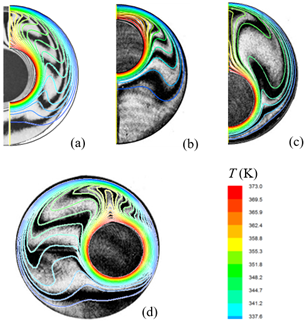Figure 7. Comparison of the current numerical temperature patterns (colored solid lines) with the experimental interferograms (black and white image) of Kuehn and Goldstein  for both concentric and eccentric annular cylinder

## 8.png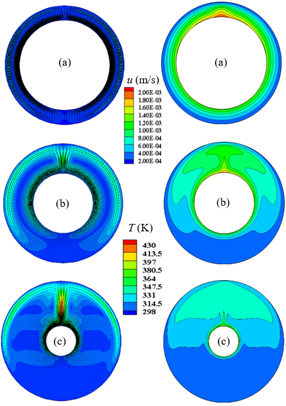Figure 8. Contours of velocity with relevant vectors (left) and temperature fields (right) corresponding to the (a) R* = 0.75, (b) R* = 0.5 and (c) R* = 0.25

Moreover, according to this formed vortex at the upper half of the annulus, the highest heat flux of the confined fluid is created. In a boundary layer adjacent to the outer cylinder, the warm fluid then travels towards the bottom of the annulus. A temperature inversion occurs in the part of the area between the two boundary layers, the fluid being colder near the cold surface than that closer to the hot surface.

3.2 Concentric annulus cylinders

Figure 9 exhibits the average Nusselt number around the surface of the inner rotating cylinder against the Taylor number with various aspect ratios. The examined range of Taylor number and annular aspect ratios are Ta = 2 - 44783 and R* = 0.25, 0.5 and 0.75, respectively, whereas the Prandtl number of the unused engine oil is kept constant at 8960.28. As mentioned before, the inner rotating cylinder is heated whilst the outer stationary one is kept at constant room temperature. Therefore, the convective heat transfer occurs from the inner to the outer cylinder across the confined fluid between them. Generally, the Nusselt number increases with increasing Taylor number and decreasing the aspect ratio. The value of R* = 0.25 recorded the highest values of the Nusselt number. It is worth mentioning that beyond the value of approximately 1700 for the Taylor number, a significant increase in the Nusselt number can be shown, and this is because of the creation of a secondary vortex by the centrifugal force. Furthermore, for the case of concentric annular cylinder with internal rotating cylinder, the percentage values of heat transfer enhancement compared to free convection heat transfer in the concentric annulus (Ω = 0) corresponding to the R* = 0.75, R* = 0.5 and R* = 0.25 are approximately (22% at 500 rpm - 65% at 3000 rpm), (50% at 500 rpm - 87% at 3000 rpm) and (60% at 500 rpm - 80% at 3000 rpm), respectively.

The present numerical data of Nusselt number was compared with the correlation formula ($\overline{N u}=0.22 T a^{0.25} \operatorname{Pr}^{0.3}$) obtained from the experimental results of Aoki’s and his co-workers . For R* = 0.25 and 0.5, the comparison shows good agreement between them. However, the values of Nusselt number of R* = 0.75 slightly deviates from Aoki’s formula. The justification behind this deviation is the annular gap width (radial clearance) for R* = 0.75 is out of the range of gap width used in the experiments of Aoki and his co-workers. Finally, the experimental study executed by Tachibana et al.  ($\overline{N u}=0.42(T a \times P r)^{0.25}$) also considers the influence of Prandtl number but, as with Aoki et al. , they do not completely consider the aspect ratio effects by explicitly integrating them into their experimental correlation.

Figure 10 depicts the numerical surface temperature (Ts) of the inner rotating cylinder versus Taylor number using three different aspect ratios. It can be seen that the surface temperature gradually decreases with increasing Taylor number. Also, with decreasing aspect ratio, a gradual decrease in the values of surface temperature is shown.

The relation between the moment coefficient (Cmc) and Taylor number is illustrated in Figure 11, for various aspect ratios in the range of internal cylinder rotational speed of 500 - 3000 rpm. The rotational Reynolds number has been implicitly calculated in Eq. (15) from Eq. (16) in order to evaluate the moment coefficient. The computed range of rotational Reynolds number is from 63.25 to 141.5 corresponding to the angular velocity from 500 to 3000 rpm, respectively.

## 9.png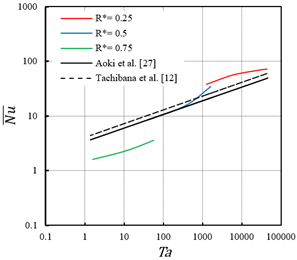Figure 9. Values of mean Nusselt number around the surface of inner rotating cylinder against Taylor number with various aspect ratios

## 10.png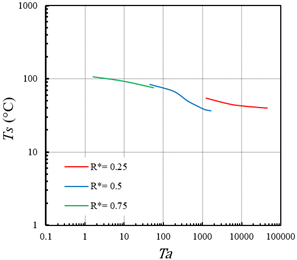Figure 10. Temperature of the surface of inner rotating cylinder against Taylor number with various aspect ratios

In general, Figure 11 shows that the moment coefficient decreases with the rising Taylor number. In spite of the significant variation in the aspect ratio, the decreasing trend of the moment coefficient for all aspect ratios is similar. It is worthwhile to mention that the torque is determined from the computational simulation by quantifying the shear stress at the wall of the internal rotating cylinder where the transmitted torque equals (τAsRin) for the circumferential flow within the concentric annular cylinder. As shown in Figure 11, the data of the moment coefficient obtained from the numerical simulation is compared with the data of the moment coefficient resulted from solving the linear theory (Eq. (15)). The numerical results of the moment coefficient are well consistent with the relation found from the linear theory (Eq. (15)). For all aspect ratios, the maximum mean deviation of Eq. (15) from numerical data is nearly ±7.5%.

Moreover, the data from the experimental correlation of Bilgen and Boulos  is also added for comparison. The values of the moment coefficient calculated from the empirical correlation equation ($C_{m c}=10 \times\left(\frac{R_{\text {out }}-R_{\text {in }}}{R_{\text {in }}}\right)^{0.3} R e_{\theta}^{-1}$) show good agreement with the analytical data (Eq. (14)) for both 0.5 and 0.75 aspect ratios, while for 0.25 aspect ratio, the maximum deviation is approximately 19%. Moreover, they reported that the maximum averaged deviation of Eq. (15) from their experimental data was ±5.8% .

## 11.png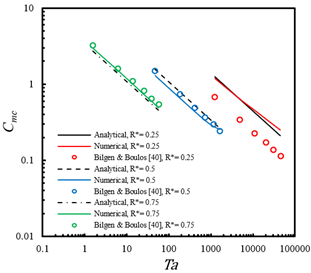Figure 11. The variation of moment coefficients for a rotating cylinder as a function of the Taylor numbers with various aspect ratios

As discussed prior in Figures 9, 10 and 11, the aspect ratio of the annular cylinder, possesses a significant influence on convection heat transfer in the annular gap. In the following investigated cases, the width of the annular gap has been changed to achieve different aspect ratios (R* = 0.75, 0.5 and 0.25). The structural characteristics of flow in the annular gap are visualized via the r-θ plane of velocity contours and vectors corresponding to the low and high Taylor numbers (500 and 3000 rpm) for three various aspect ratios as depicted in Figure 12. It is obvious from Figure 12 (a, b and c), the intensity of velocity vectors concentrates at the perimeter of the inner rotating cylinder and these vectors vanish toward the outer stationary cylinder. Additionally, the strength of circumferential vortices (so-called Taylor's vortices) in the vicinity of the inner rotating cylinder continues to increase with the increase in the rotation speed (500 - 3000 rpm). The development in the annular gap flow caused by the change in the aspect ratio and rotation speed has resulted in a significant effect on the fluid flow patterns in the annular gap as shown in Figure 12. Therefore, this can promote the mixing process between the hot surface of the rotating cylinder and the confined fluid in the annular gap.

It is expected that the temperature field is also influenced due to the appearance of vortices in the vicinity of the inner cylinder as illustrated in Figure 13. It can be indicated that the heat dissipation from the internal rotating cylinder rises with increasing Taylor number and decreasing aspect ratio. The homogeneous temperature field is approximately obtained in the annular gap flow at 0.5 and 0.25 aspect ratios and a high rotation speed of 3000 as shown in Figure 13 (a and b). On the other hand, the remarkable gradient in the fluid temperature at a high aspect ratio (R* = 0.75) is noticed due to the restricted fluid movement in the narrow annular gap, which in turns reduces the fluid mixing efficiency.

## 12.png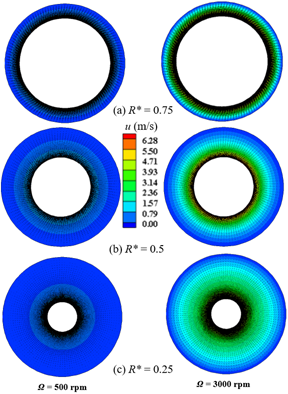Figure 12. Velocity contours with relevant vectors corresponding to the (a) R* = 0.75, (b) R* = 0.5 and (c) R* = 0.25 for rotation speeds 500 and 3000 rpm

## 13.png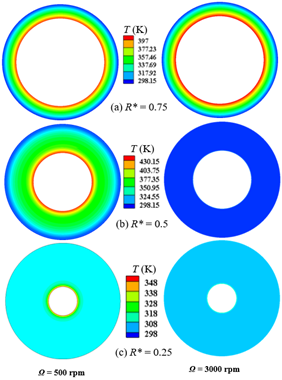Figure 13. Patterns of temperature distribution for rotation speeds 500 and 3000 rpm corresponding to the (a) R* = 0.75, (b) R* = 0.5 and (c) R* = 0.25

3.3 Eccentric annulus cylinders

To obtain the optimum design that enhances the hydrothermal performance in an eccentric annular cylinder, 21 runs to five levels of variables (used parameters) are employed in response surface methodology. The selected parameters and their levels (low (-1.414), central (0), and high (+1.414) levels) are listed in Table 3. According to the face-centred central composite design fashion, the intervals are divided into four equal sub-intervals. The inclination angle of the inner rotating cylinder, θ (deg), (A) and the eccentricity, E (cm), (B) are adopted as experimentation parameters in this investigation at R* = 0.25 and Ta = 3.11×104. Basically, response surface methodology (RSM) is a statistical-mathematical method, which utilized for an empirical model constructing, i.e., developing a mathematical model of the second-order response surface involving with best fittings. In this response surface method, the relationships between several explanatory variables (inputs) and one or more response parameters (outputs) are predicted [43-45]. A robust software of Design-Expert 12 was employed in this study to analyze the numerical data, proceed with RSM and obtain two-dimensional and three-dimensional response graphs.

Table 3. Design range of input variables (θ and E) along with their intervals

 Input variables Coded symbol Levels (design spaces) -1.414 -1 0 1 1.414 The inclination angle of the inner cylinder (θ, deg) A 0 45 90 135 180 Eccentricity (E, cm) B 0.5 1 1.5 2 2.5

Table 4. Design variables of tests according to the central composite design method for the Cmc, $\overline{N u}$  and Ts of the eccentric annular cylinder

 Exp. No. Coded values Real values Responses A B θ (deg) E (cm) Cmc $\overline{N u}$ Ts (°C) 1 1.414 0 180 1.5 0.4112 75.34 39.6 2 0 0 90 1.5 0.4112 75.34 39.6 3 -1 1 45 2 0.6371 88.7 38 4 1 1 135 2 1.1900 96.34 37.37 5 1.414 0 180 1.5 0.4112 75.34 39.6 6 -1.414 0 0 1.5 0.4112 75.32 39.6 7 1 -1 135 1 0.6144 78.98 38.6 8 -1 -1 45 1 0.4191 74.57 39.3 9 0 0 90 1.5 0.4112 75.34 39.6 10 1 -1 135 1 0.6144 78.98 38.6 11 0 -1.414 90 2.5 0.3347 93.97 37.95 12 -1 -1 45 1 0.4191 74.57 39.3 13 1 1 135 2 1.1900 96.34 37.37 14 0 -1.414 90 2.5 0.3347 93.97 37.95 15 0 0 90 1.5 0.4112 75.34 39.6 16 0 1.414 90 2.5 0.7685 93.97 37.95 17 -1.414 0 0 1.5 0.4112 75.32 39.6 18 -1 1 45 2 0.6371 88.7 38 19 0 0 90 1.5 0.4112 75.34 39.6 20 0 1.414 90 2.5 0.7685 93.97 37.95 21 0 0 90 1.5 0.4112 75.34 39.6

In this investigation, the variables comprise the inclination angle of the inner rotating cylinder (A) and eccentricity (B). Also, the moment coefficient, the average Nusselt number and surface temperature of the eccentric inner rotating cylinder are selected as response parameters. Thus, according to the previously defined conditions, statistical analysis of the final 21 runs (numerical experiments) is implemented. Table 4 summarizes the information of coded values and real values along with responses for the input variables and the output response parameters. In Table 4, the coded values of the parameters are displayed in columns A and B, where the values of +1,414, 0, and -1,414, respectively, correspond to the high, middle and low levels. Moreover, the corresponding real values for each independent variable are tabulated in the next two columns of the inclination angle of the inner rotating cylinder, θ (deg), and eccentricity, E (cm). The values of moment coefficient, average Nusselt number and surface temperature are inserted under the column of responses.

The predicted values of moment coefficient, average Nusselt number and surface temperature as a function of the inclination angle of the inner rotating cylinder (A) and eccentricity (B) are depicted in Figure 14 as a 3D surface and a 2D contour.

## 1414.jpgFigure 14. Variations of (a) the moment coefficient, (b) the average Nusselt number and (c) surface temperature as a 3D and a 2D contour with E and θ

With Figure 14 (a), it can be noticed that variables of inclination angle (E) and eccentricity (θ) have a significant effect on the moment coefficient (Cmc). By increasing the E and θ, the moment coefficient increases and its highest value (Cmc ≈ 1.06) is observed in levels of (+1.414) and (+1.414), respectively. While the lowest value of moment coefficient (Cmc ≈ 0.338) in (−1.414) and (−1.414) for the E and θ, respectively. The influences of the eccentricity and inclination angle on the average Nusselt number $(\overline{N u})$ are illustrated in Figure 14 (b). It is indicated that the maximum value of the average Nusselt number $(\overline{N u} \approx 105)$ occurs near the highest level (+1.414) of both E and θ whilst, it is the lowest $(\overline{N u} \approx 72)$ at (−0.707) and (0) for the E and θ, respectively. Furthermore, the effect of inclination angle on augmentation of the average Nusselt number is more visible with the lowest value of eccentricity. Moreover, the impacts of the inclination angle and eccentricity on the surface temperature (Ts) are displayed in Figure 14 (c). It is observed that the maximum surface temperature (Ts ≈ 40℃) takes place at the middle levels (−0.707) for the E and (0) for the θ. It can also be noticed that the minimum value of surface temperature (Ts ≈ 37℃) occurs at the highest values (+1.414) of both E and θ.

Contours and vectors of the cross-flow velocity distribution and temperature fields in an annulus with 180° inclination angle and 2.5 cm eccentricity at 2500 rpm rotation speed are displayed in Figure 15. As predicted by the statistical software DX-12, the maximum average Nusselt number and minimum surface temperature with the moderate value of moment coefficient (Cmc) are obtained at 2.5 cm eccentricity and 180° inclination angle, which are considered the optimum-estimated parameters of the eccentric annular gap. The predicted values from DX-12 and the numerical data for the Cmc, $\overline{N u}$ and Ts of the eccentric annular cylinder are listed in Table 5 where the accuracy between them is approximately 99%.

Table 5. Comparison between the predicted DX-12 and CFD data for the Cmc, $\overline{N u}$ and Ts of eccentric annular cylinder at θ=180° and E = 2.5cm

 Parameters Predicted DX CFD Data Accuracy (%) Cmc 0.338 0.340 99.0 $\overline{N u}$ 104.9 105.5 99.4 Ts (°C) 36.9 37.2 99.2

## 15.png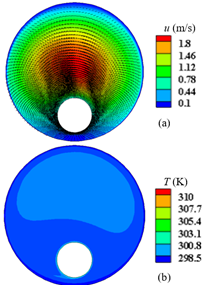Figure 15. Contours of velocity with relevant vectors (a) and temperature fields (b) for the optimum eccentric annular cylinder at θ = 180°, E = 2.5 cm and Ω = 2500 rpm

As can be noticed from the velocity contours and vectors in Figure 15 (a), at the eccentricity of 2.5 cm and inclination angle of 180°, the entire annular gap is dominated by the reverse flow where the confined fluid circulates in the opposite direction to the inner cylinder rotation. Thus, a high-temperature distribution occurs in the widening annular gap close to the wall of the outer cylinder (see Figure 15 (b)). Moreover, at the narrowest gap, the fluid turns and flows back along the stationary surface of the outer cylinder. This arises a region of cold fluid within the smallest width of the annular gap associated with high eccentricity.

According to Webb's criteria (Performance Evaluation Criteria, PEC) , the thermal-hydraulic performance evaluation for the case of optimum-estimated parameters at inclination angle, θ = 180o and eccentricity, E = 2.5 cm and the case of the lowest average Nusselt number, which is at 90° inclination angle and 0.5 cm eccentricity, equals to 1.97. In other words, the thermal-hydraulic enhancement is 97 percent improved.

$P E C=\frac{\overline{N u}_{\theta}=180^{\circ}, E=2.5 / \overline{N u}_{\theta}=90^{\circ}, E=0.5}{\left(C m C_{\theta}=180^{\circ}, E=2.5 / C m C_{\theta}=90^{\circ}, E=0.5\right)^{\frac{1}{3}}}$      (19)

4. Conclusions

Characteristics of convective heat transfer in a concentric and an eccentric annular cylinder with a heated internal rotating cylinder were numerically studied. The three-dimensional computational simulation was developed using the well-known commercial software ANSYS FLUENT. Also, a sensitivity analysis was executed via response surface methodology (RSM) to predict the optimum design that enhances the hydrothermal performance in an eccentric annular cylinder.

For the concentric annular cylinder case, the Nusselt number increases with the rising Taylor number and decreasing the aspect ratio. However, the moment coefficient and surface temperature gradually decrease with increasing Taylor number and with decreasing aspect ratio. A significant increase in Nusselt number can be seen beyond the value of approximately 1700 for the Taylor number, and this is because of the centrifugal force producing secondary vortices.

For the eccentric annular cylinder case, the discussed results above pointed out that variations of inclination angle (θ) and eccentricity (E) of the inner rotating cylinder have a significant impact on the moment coefficient, the average Nusselt number and the surface temperature. As predicted by the statistical software DX-12, the maximum mean Nusselt number and minimum surface temperature with the moderate value of moment coefficient are obtained at 2.5 cm eccentricity and 180° inclination angle, which are considered the optimum-estimated parameters of the eccentric annular gap by 97% enhanced. Additionally, the numerical simulations of annular oil flow and thermal fields have presented valuable information to substantiate the mechanism of convective heat transfer associated with various arrangements of heated inner rotating cylinder and aspect ratio. The variable thermophysical properties of engine oil are currently underway as the second part of a two-part examination.

References

 Greenspan, H.P. (1968). The Theory of Rotating Fluid. Cambridge University Press, London, 77(2): 247. https://doi.org/10.1086/627430

 Couette, M. (1887). Oscillations tournantes d’un solide de révolution en contact avec un fluide visqueux. Compt. Rend. Acad. Sci. Paris, 105: 1064-1067.

 Couette, M. (1890). Etudes sur le frottement des liquides. Ann. Chim. Phys., 6(21): 433-510.

 Mallock, A. (1889). IV. Determination of the viscosity of water. Proceedings of the Royal Society of London, 45(273-279): 126-132. https://doi.org/10.1098/rspl.1888.0081

 Mallock, A. (1896). III. Experiments on fluid viscosity. Philosophical Transactions of the Royal Society of London. Series A, Containing Papers of a Mathematical or Physical Character, (187): 41-56. https://doi.org/10.1098/rsta.1896.0003

 Taylor, G.I. (1923). VIII. Stability of a viscous liquid contained between two rotating cylinders. Philosophical Transactions of the Royal Society of London. Series A, Containing Papers of a Mathematical or Physical Character, 223(605-615): 289-343. https://doi.org/10.1098/rsta.1923.0008

 Di Prima, R.C., Swinney, H.L. (1981). Instabilities and transition in flow between concentric rotating cylinders. In Hydrodynamic Instabilities and the Transition to Turbulence, pp. 139-180. https://doi.org/10.1007/3-540-13319-4_16

 Dou, H.S., Khoo, B.C., Yeo, K.S. (2008). Instability of Taylor–Couette flow between concentric rotating cylinders. International Journal of Thermal Sciences, 47(11): 1422-1435. https://doi.org/10.1016/j.ijthermalsci.2007.12.012

 Andereck, C.D., Liu, S.S., Swinney, H.L. (1986). Flow regimes in a circular Couette system with independently rotating cylinders. Journal of Fluid Mechanics, 164: 155-183.‏ https://doi.org/10.1017/S0022112086002513

 Esser, A., Grossmann, S. (1996). Analytic expression for Taylor–Couette stability boundary. Physics of Fluids, 8(7): 1814-1819. https://doi.org/10.1063/1.868963

 Bjorklund, I.S., Kays, W.M. (1959). Heat transfer between concentric rotating cylinders. Journal of Heat Transfer, 81(3): 175-183. https://doi.org/10.1115/1.4008173

 Tachibana, F., Fukui, S., Mitsumura, H. (1960). Heat transfer in an annulus with an inner rotating cylinder. Bulletin of JSME, 3(9): 119-123. https://doi.org/10.1299/jsme1958.3.119

 Becker, K.M., Kaye, J. (1962). The influence of a radial temperature gradient on the instability of fluid flow in an annulus with an inner rotating cylinder. Journal of Heat Transfer, 84(2): 106-110. https://doi.org/10.1115/1.3684306

 Guo, Z.Y., Zhang, C.M. (1992). Thermal drive in centrifugal fields—mixed convection in a vertical rotating cylinder. International Journal of Heat and Mass Transfer, 35(7): 1635-1644. https://doi.org/10.1016/0017-9310(92)90134-E

 Kuehn, T.H., Goldstein, R.J. (1976). An experimental and theoretical study of natural convection in the annulus between horizontal concentric cylinders. Journal of Fluid Mechanics, 74(4): 695-719.‏ https://doi.org/10.1017/S0022112076002012

 Kuehn, T.H., Goldstein, R.J. (1978). An experimental study of natural convection heat transfer in concentric and eccentric horizontal cylindrical annuli. Journal of Heat Transfer, 100(4): 635-640. https://doi.org/10.1115/1.3450869

 Shareef, A.J., Abed, W.M., Najeeb, A.A. (2010). Natural convection heat transfer in horizontal concentric annulus between outer cylinder and inner flat tube. Anbar Journal of Engineering Science, 3(2): 31-45.

 Dawood, H.K., Mohammed, H.A., Sidik, N.A.C., Munisamy, K.M., Wahid, M.A. (2015). Forced, natural and mixed-convection heat transfer and fluid flow in annulus: A review. International Communications in Heat and Mass Transfer, 62: 45-57. https://doi.org/10.1016/j.icheatmasstransfer.2015.01.006

 Kareem, M.K., Abed, W.M., Dawood, H.K. (2020). Numerical simulation of hydrothermal behavior in a concentric curved annular tube. Heat Transfer, 49(5): 2494-2520. https://doi.org/10.1002/htj.21732

 Maron, D.M., Cohen, S. (1991). Hydrodynamics and heat/mass transfer near rotating surfaces. In Advances in Heat Transfer, 21: 141-183.‏ https://doi.org/10.1016/S0065-2717(08)70335-6

 Fénot, M., Bertin, Y., Dorignac, E., Lalizel, G. (2011). A review of heat transfer between concentric rotating cylinders with or without axial flow. International Journal of Thermal Sciences, 50(7): 1138-1155.‏ https://doi.org/10.1016/j.ijthermalsci.2011.02.013

 Luke, G.E. (1926). Surface heat transfer in electric machines with forced air flow. Transactions of the American Institute of Electrical Engineers, 45: 1036-1047. https://doi.org/10.1109/JAIEE.1926.6537842

 Hoseason, D.B. (1931). The cooling of electrical machines. Journal of the Institution of Electrical Engineers, 69(409): 121-143. https://doi.org/10.1049/jiee-1.1931.0001

 Gazley Jr, C. (1952). Air-Gap Heat Transfer in Rotating Electric Machines. General Electric Report No. DF 52GL156.

 Gazley Jr, C. (1958). Heat-transfer characteristics of rotational and axial flow between concentric cylinders. Transactions of the ASME, 1: 79-90.

 Tachibana, F., Fukui, S. (1964). Convective heat transfer of the rotational and axial flow between two concentric cylinders. Bulletin of JSME, 7(26): 385-391. https://doi.org/10.1299/jsme1958.7.385

 Aoki, H., Nohira, H., Arai, H. (1967). Convective heat transfer in an annulus with an inner rotating cylinder. Bulletin of JSME, 10(39): 523-532. https://doi.org/10.1299/jsme1958.10.523

 Becker, K.M., Kaye, J. (1962). Measurements of diabatic flow in an annulus with an inner rotating cylinder. Journal of Heat Transfer, 84(2): 97-104. https://doi.org/10.1115/1.3684335

 Ball, K.S., Farouk, B. (1987). On the development of Taylor vortices in a vertical annulus with a heated rotating inner cylinder. International Journal for Numerical Methods in Fluids, 7(8): 857-867. https://doi.org/10.1002/fld.1650070806

 Ball, K.S., Farouk, B., Dixit, V.C. (1989). An experimental study of heat transfer in a vertical annulus with a rotating inner cylinder. International Journal of Heat and Mass Transfer, 32(8): 1517-1527. https://doi.org/10.1016/0017-9310(89)90073-2

 Char, M.I., Hsu, Y.H. (1998). Numerical prediction of turbulent mixed convection in a concentric horizontal rotating annulus with low-Re two-equation models. International Journal of Heat and Mass Transfer, 41(12): 1633-1643.‏ https://doi.org/10.1016/S0017-9310(97)00304-9

 Lee, Y.N., Minkowycz, W.J. (1989). Heat transfer characteristics of the annulus of twocoaxial cylinders with one cylinder rotating. International Journal of Heat and Mass Transfer, 32(4): 711-722. https://doi.org/10.1016/0017-9310(89)90218-4

 Ali, M.A., El-Maghlany, W.M., Eldrainy, Y.A., Attia, A. (2018). Heat transfer enhancement of double pipe heat exchanger using rotating of variable eccentricity inner pipe. Alexandria Engineering Journal, 57(4): 3709-3725. https://doi.org/10.1016/j.aej.2018.03.003

 Abou-Ziyan, H.Z., Helali, A.H.B., Selim, M.Y. (2016). Enhancement of forced convection in wide cylindrical annular channel using rotating inner pipe with interrupted helical fins. International Journal of Heat and Mass Transfer, 95: 996-1007. https://doi.org/10.1016/j.ijheatmasstransfer.2015.12.066

 Yassin, M.A., Shedid, M.H., Abd El-Hameed, H.M., Basheer, A. (2018). Heat transfer augmentation for annular flow due to rotation of inner finned pipe. International Journal of Thermal Sciences, 134: 653-660. https://doi.org/10.1016/j.ijthermalsci.2018.05.033

 Childs, P.R. (2010). Rotating flow. Elsevier.‏ https://doi.org/10.1016/C2009-0-30534-6

 Tannehill, J.C., Pletcher, R.H., Anderson, D.A. (2011). Computational Fluid Mechanics and Heat Transfer, 3rd Edition, CRC Press.

 Bergman, T.L., Incropera, F.P., Lavine, A.S., Dewitt, D.P. (2011). Introduction to Heat Transfer. John Wiley & Sons.

 Donnelly, R.J., Simon, N.J. (1960). An empirical torque relation for supercritical flow between rotating cylinders. Journal of Fluid Mechanics, 7(3): 401-418. https://doi.org/10.1017/S0022112060000177

 Bilgen, E., Boulos, R. (1973). Functional dependence of torque coefficient of coaxial cylinders on gap width and Reynolds numbers. Trans. ASME, Journal of Fluids Engineering, 95: 122-126. https://doi.org/10.1115/1.3446944

 Cengel, Y. (2014). Heat and Mass Transfer: Fundamentals and Applications. McGraw-Hill Higher Education.

 Inc, A. (2011). ANSYS Fluent V 14.5 User’s Guide, Fluent Inc, Lebanon NH.

 Rashidi, S., Bovand, M., Esfahani, J.A. (2017). Sensitivity analysis for entropy generation in porous solar heat exchangers by RSM. Journal of Thermophysics and Heat Transfer, 31(2): 390-402. https://doi.org/10.2514/1.T5003

 Diel, C.L., Canevesi, R.L.S., Zempulski, D.A., Awadallak, J.A., Borba, C.E., Palú, F., Silva, E.A. (2016). Optimization of multiple-effect evaporation in the pulp and paper industry using response surface methodology. Applied Thermal Engineering, 95: 18-23. https://doi.org/10.1016/j.applthermaleng.2015.10.136

 Silva, V.B., Rouboa, A. (2012). Optimizing the DMFC operating conditions using a response surface method. Applied Mathematics and Computation, 218(12): 6733-6743. https://doi.org/10.1016/j.amc.2011.12.039

 Webb, R.L. (1981). Performance evaluation criteria for use of enhanced heat transfer surfaces in heat exchanger design. International Journal of Heat and Mass Transfer, 24(4): 715-726. https://doi.org/10.1016/0017-9310(81)90015-6